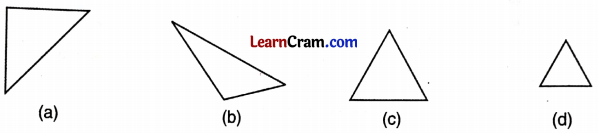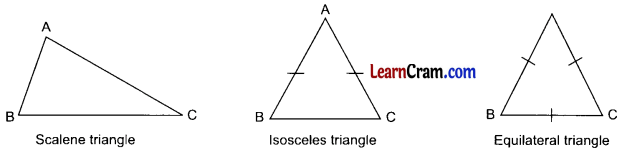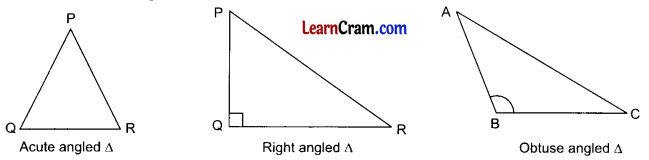# DAV Class 6 Maths Chapter 12 Worksheet 1 Solutions

The DAV Maths Book Class 6 Solutions and DAV Class 6 Maths Chapter 12 Worksheet 1 Solutions of Triangles offer comprehensive answers to textbook questions.

## DAV Class 6 Maths Ch 12 WS 1 Solutions

Question 1.
Draw any X triangle XYZ and name its sides, angles and vertices.
In ΔXYZ,
Sides are XY, YZ and ZX
Angles are ∠XYZ, ∠YZX and ∠YXZ
Vertices are X, Y and Z

Question 2.
How many triangles are there in each of the following figures?(a) 3 triangles
(b) 5 triangles
(c) 8 triangles
(d) 8 trianglesQuestion 3.
Identify the following triangles on the basis of their angles.(a) Right angled triangle
(b) Obtuse angled triangle
(c) Acute angled triangle
(d) Acute angled triangle

Question 4.
Can you construct a triangle with angles of measure 70°, 40°, 60°? Why or why not?
Sum of the angles
= 70° + 40° + 60° = 170°
No, we cannot construct this triangle as the sum of all the angles of a triangle is not 180°.

Question 5.
Can you construct a triangle using line segments of the following lengths?
(a) 7 cm, 3 cm, 12 cm
(b) 6 cm, 5 cm, 11 cm
(a) As per the property of triangle, the lengths of any two sides of a triangle must be greater than the length of the third side.
Here 3 cm + 7 cm = 10 cm
10 cm < 12 cm
Hence, it is not possible to construct this triangle.

(b) Here 5 cm + 6 cm =11 cm
11 cm =11 cm
The sum of two sides is equal to the third side of the triangle.
Hence, the triangle is not possible to be constructed.

Question 6.
One of the angles of a right angled triangle is 40°.
Find the other angles of the triangle.Let us take a right angled triangle ABC, in which ZB = 90°
∠A + ∠C = 90°
[Remaining angles of the triangle]
40° + ∠C = 90°
∠C = 90° – 40° = 50°
Hence the third angle is 50°.Question 7.
Two angles of a triangle measure 30° and 55° respectively. Find the measures of the third angle. Also state what type of triangle is it?
Sum of the three angles of a triangle = 180°
Measure of two angles = 30° and 55°
∴ Measure of the third angle
= 180°-(30°+ 55°)
= 180° – 85°
= 95°
95° > 90°
Hence the triangle is obtuse angled triangle.

Question 8.
Write ‘True’ or ‘False’ for the following statements:
(a) The sum of three angles of a triangle is always equal to 180°.
True

(b) A triangle can have two of its angles greater than 90°.
False

(c) Equilateral triangle has all of its three sides equal in length.
True

(d) One angle of a right angled triangle is always equal to 90°.
True

(e) A triangle whose all the three sides are different in length is called an isosceles triangle.
False

### DAV Class 6 Maths Chapter 12 Worksheet 1 Notes

A figure enclosed by three line segments is called a triangle. It has 3 angles and 3 sides.

Types of Triangle
1. Scalene triangle:
The triangle having all the sides of different lengths is called scalene triangle. In the given figure, AB ≠ BC ≠ AC.

2. Isosceles triangle:
The triangle whose any two sides are equal is called isosceles triangle.
In the given figure, AB = AC.

3. Equilateral triangle:
The triangle whose all sides are equal is called equilateral triangle. In the given figure, AB = BC = CA4. Acute angled triangle: The triangle in which each angle is acute is called acute angled triangle.
Here, in ΔPQR, all angles are acute.

5. Right angled triangle: The triangle whose one angle is 90° is called right angled triangle.
Here, in ΔPQR, ∠Q = 90°.

6. Obtuse angled triangle: The triangle in which only one angle is obtuse is called obtuse angled triangle.Here, ∠B is obtuse angle.